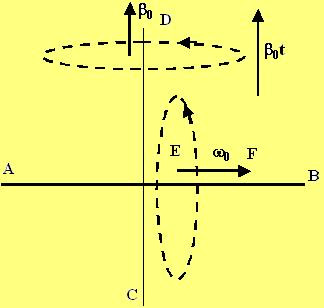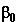## Saturday, August 4, 2007

### Irodov Problem 1.58At first the object just turns around the stationary axis AB at and angular velocityoriented along AB. Then, AB itself turns around the axis CD with a constant angular acceleration- the acceleration vector is directed along CD. Now at any instant, the the object is also rotating about CD with an angular velocity vectordirected along CD. The net angular velocity vector (obtained by the addition of the two vectors) magnitude is thus given by,.

The object experience two kinds of angular accelerations, i) along CD with magnitudeand ii) the other because the angular velocity vector EF itself rotates about CD.

The angle that the vector EF has rotated by time t is given by. Thus, the angular velocity of the particle as a function of time is given by,This is the last of the problems in the kinematics section of I.E. Irodov. Now we shall move on to the fundamental equation of dynamics.